MONOTONE Statement
MONOTONE <method < ( < imputed < = effects> >   </ options> ) > >
<...method < ( < imputed < = effects> >   </ options> ) > >
;

The MONOTONE statement specifies imputation methods for data sets with monotone missingness. You must also specify a VAR statement, and the data set must have a monotone missing pattern with variables ordered in the VAR list.

Table 56.4 summarizes the options available for the MONOTONE statement.

Table 56.4 Summary of Imputation Methods in MONOTONE Statement

Option

Description

Specifies the discriminant function method

Specifies the logistic regression method

Specifies the propensity scores method

Specifies the regression method

Specifies the predictive mean matching method

For each method, you can specify the imputed variables and, optionally, a set of the effects to impute these variables. Each effect is a variable or a combination of variables preceding the imputed variable in the VAR statement. The syntax for specification of effects is the same as for the GLM procedure. See Chapter 41, The GLM Procedure, for more information.

One general form of an effect involving several variables is

X1X2ABCD Ewhere A, B, C, D, and E are classification variables and X1 and X2 are continuous variables.

If no covariates are specified, then all preceding variables are used as the covariates. That is, each preceding continuous variable is used as a regressor effect, and each preceding classification variable is used as a main effect. For the discriminant function method, only the continuous variables can be used as covariate effects.

When a method for continuous variables is specified without imputed variables, the method is used for all continuous variables in the VAR statement that are not specified in other methods. Similarly, when a method for classification variables is specified without imputed variables, the method is used for all classification variables in the VAR statement that are not specified in other methods.

When a MONOTONE statement is used without specifying any methods, the regression method is used for all continuous variables and the discriminant function method is used for all classification variables. The preceding variables of each imputed variable in the VAR statement are used as the covariates.

With a MONOTONE statement, the variables are imputed sequentially in the order given by the VAR statement. For a continuous variable, you can use a regression method, a regression predicted mean matching method, or a propensity score method to impute missing values.

For a nominal classification variable, you can use a discriminant function method to impute missing values without using the ordering of the class levels. For an ordinal classification variable, you can use a logistic regression method to impute missing values by using the ordering of the class levels. For a binary classification variable, either a discriminant function method or a logistic regression method can be used.

Note that except for the regression method, all other methods impute values from the observed observation values. You can specify the following methods in a MONOTONE statement.

DISCRIM <( imputed < = effects> <options> ) >

specifies the discriminant function method of classification variables. Only the continuous variables are allowed as covariate effects. The available options are DETAILS, PCOV=, and PRIOR=. The DETAILS option displays the group means and pooled covariance matrix used in each imputation. The PCOV= option specifies the pooled covariance used in the discriminant method. Valid values for the PCOV= option are as follows:

FIXED

uses the observed-data pooled covariance matrix for each imputation.

POSTERIOR

draws a pooled covariance matrix from its posterior distribution.

The default is PCOV=POSTERIOR. See the section Monotone and FCS Discriminant Function Methods for a detailed description of the method.

The PRIOR= option specifies the prior probabilities of group membership. Valid values for the PRIOR= option are as follows:

EQUAL

sets the prior probabilities equal for all groups.

PROPORTIONAL

sets the prior probabilities proportion to the group sample sizes.

JEFFREYS < =c >

specifies a noninformative prior,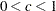. If the numberis not specified, JEFFREYS=0.5.

RIDGE < =d >

specifies a ridge prior,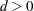. If the number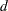is not specified, RIDGE=0.25.

The default is PRIOR=JEFFREYS. See the section Monotone and FCS Discriminant Function Methods for a detailed description of the method.

LOGISTIC <( imputed < = effects> <options> ) >

specifies the logistic regression method of classification variables. The available options are DETAILS, ORDER=, and DESCENDING. The DETAILS option displays the regression coefficients in the logistic regression model used in each imputation.

When the imputed variable has more than two response levels, the ordinal logistic regression method is used. The ORDER= option specifies the sorting order for the levels of the response variable. Valid values for the ORDER= option are as follows:

DATA

sorts by the order of appearance in the input data set.

FORMATTED

sorts by their external formatted values.

FREQ

sorts by the descending frequency counts.

INTERNAL

sorts by the unformatted values.

By default, ORDER=FORMATTED.

The option DESCENDING reverses the sorting order for the levels of the response variables.

See the section Monotone and FCS Logistic Regression Methods for a detailed description of the method.

PROPENSITY <( imputed < = effects> <options> ) >

specifies the propensity scores method of variables. Each variable is either a classification variable or a continuous variable. The available options are DETAILS and NGROUPS=. The DETAILS option displays the regression coefficients in the logistic regression model for propensity scores. The NGROUPS= option specifies the number of groups created based on propensity scores. The default is NGROUPS=5.

See the section Monotone Propensity Score Method for a detailed description of the method.

REG | REGRESSION <( imputed < = effects> <DETAILS> ) >

specifies the regression method of continuous variables. The DETAILS option displays the regression coefficients in the regression model used in each imputation.

With a regression method, the MAXIMUM=, MINIMUM=, and ROUND= options can be used to make the imputed values more consistent with the observed variable values.

See the section Monotone and FCS Regression Methods for a detailed description of the method.

REGPMM < ( imputed < = effects> < options> ) >
REGPREDMEANMATCH < ( imputed < = effects > < options > ) >

specifies the predictive mean matching method for continuous variables. This method is similar to the regression method except that it imputes a value randomly from a set of observed values whose predicted values are closest to the predicted value for the missing value from the simulated regression model (Heitjan and Little 1991; Schenker and Taylor 1996).

The available options are DETAILS and K=. The DETAILS option displays the regression coefficients in the regression model used in each imputation. The K= option specifies the number of closest observations to be used in the selection. The default is K=5.

See the section Monotone and FCS Predictive Mean Matching Methods for a detailed description of the method.

With a MONOTONE statement, the missing values of a variable are imputed when the variable is either explicitly specified in the method or implicitly specified when a method is specified without imputed variables. These variables are imputed sequentially in the order specified in the VAR statement. For example, the following MI procedure statements use the logistic regression method to impute variable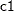from effects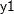,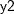, and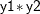first, and then use the regression method to impute variable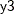from effects,, and:

```proc mi;
class c1;
var y1 y2 c1 y3;
monotone reg(y3= y1 y2 c1) logistic(c1= y1 y2 y1*y2);
run;
```

The variablesandare not imputed sinceis the leading variable in the VAR statement andis not specified as an imputed variable in the MONOTONE statement.